# How to Estimate in Maths

How to Estimate in MathsEstimation means to simplify numbers in a calculation in order to get a close answer more quickly and easily than doing the full calculation.• Estimating means to change a number to another number that is close to it.
• Estimating is used to make calculations easier and it gives us an answer that is close to the actual answer.
• 2.8 can be estimated to be close to 3.
• 5.1 can be estimated to be close to 5.
• The sum of 2.8 + 5.1 can be estimated to be 3 + 5.
• 2.8 + 5.1 = 7.9 which is the exact answer.
• This is very close to 3 + 5 = 8, our estimated answer.

Look at the digit to the right of the digit being estimated.

If this digit is 5 or more, round up.

If this digit is 4 or less, round down.• The second digit of 33 is a 3, which is ‘4 or less’.
• Therefore we round 33 down to 30 when estimating.
• The second digit of 19 is a 9, which is ‘5 or more’.
• Therefore we round 19 up to 20 when estimating.
• 33 – 19 = 14 which is the exact answer.
• 30 – 20 = 10, which is an estimated answer.#### Estimation Multiplication and Division: Video Lesson# Estimating

## Why Estimating is Important

In maths, estimation means to simplify numbers in a calculation in order to get a close answer more quickly and easily than doing the full calculation. The benefits of estimation are that it can often be completed mentally and the result can be used to check the result of a calculation.

For example, if you need to buy 5 pens for $2.99 each, it is easier to find 5 ×$3 than work out 5 × $2.99. The correct cost is$14.95 which is very close to the estimation of $15. This estimation can be completed quickly and easily in your head. ## How to Estimate to the Nearest Integer Estimating a number to the nearest integer means to find the nearest whole number to it. Look at the digit in the tenths place. If it is 5 or more, round up. If it is 4 or less round down. For example, 6.27 rounds down to 6 because there is a 2 in the tenths place.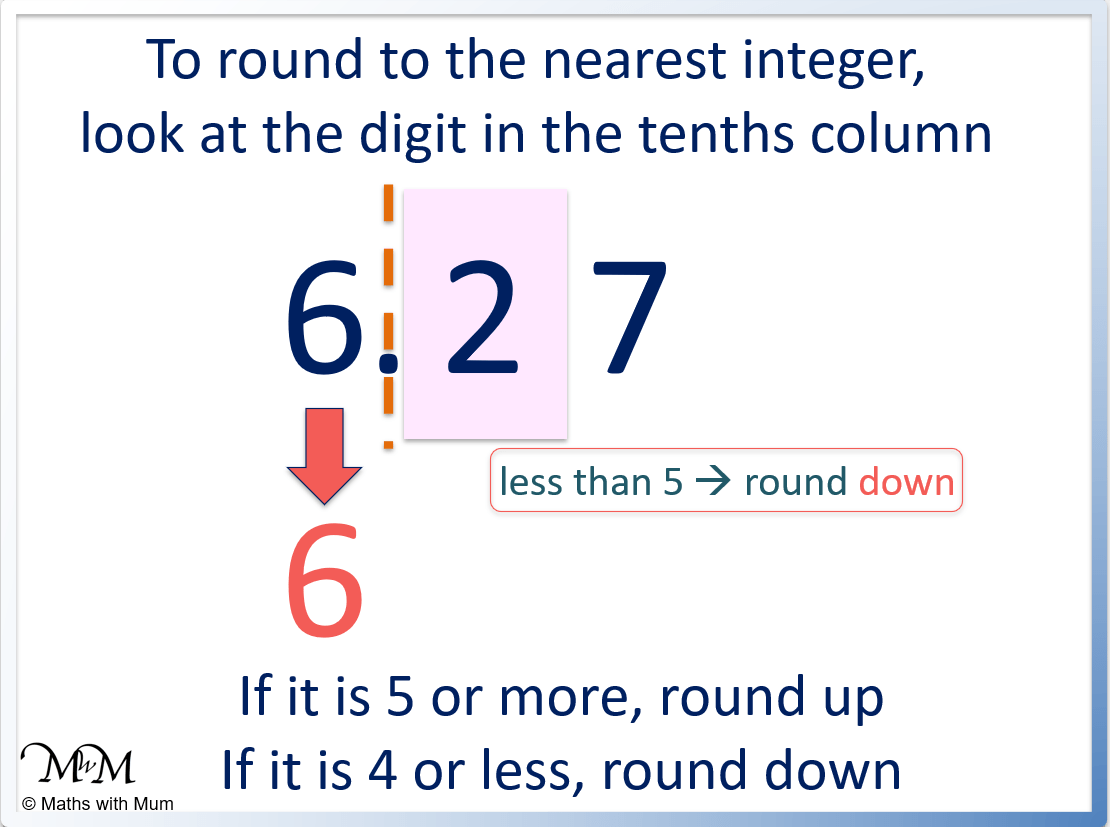An integer is a whole number. When rounding to the nearest integer, only look at the digit immediately after the decimal point in the tenths column to decide whether to round up or down. For example, estimate 2.83 to the nearest integer. The digit in the tenths column is 8. This is ‘5 or more’ and so we round up.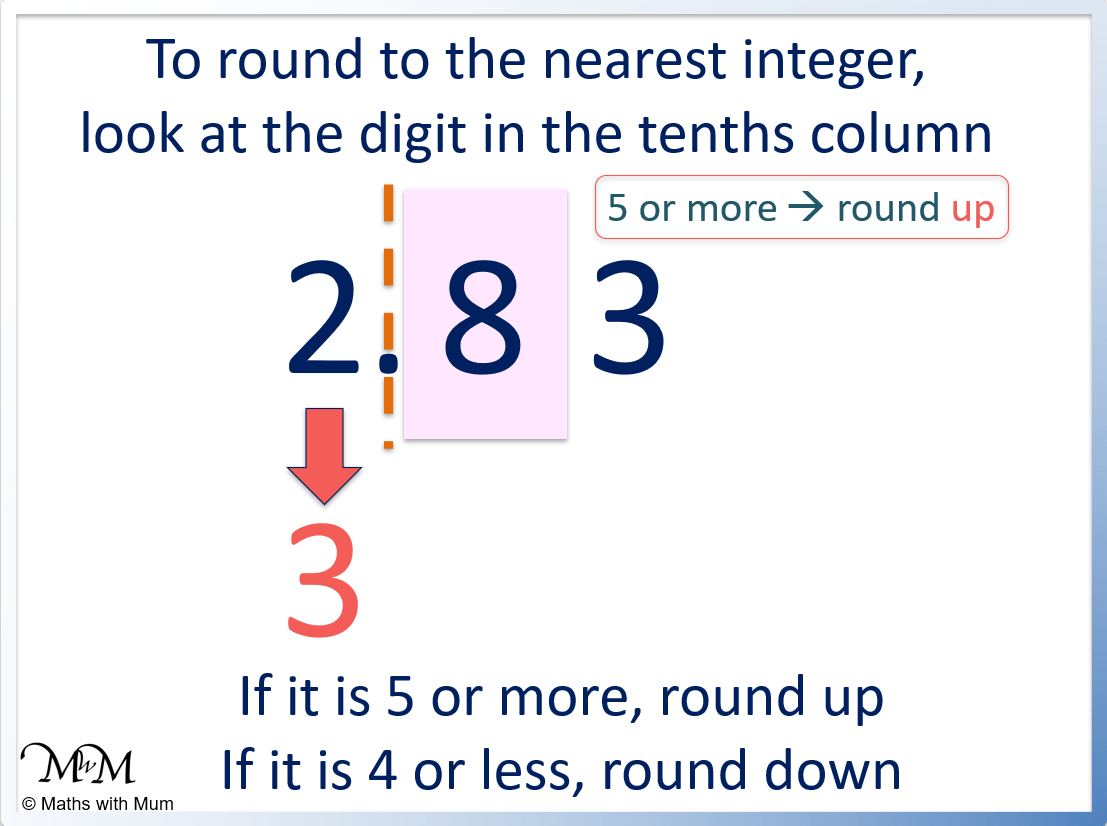We round 2.87 up to 3 which means that we estimate 2.87 to be 3. ## How to Estimate to the Nearest Ten To estimate a number to the nearest ten, look at the digit in the ones column. If it is 5 or more, round up. If it is 4 or less, round down. For example, 14 rounds down to 10 because there is a 4 in the ones column.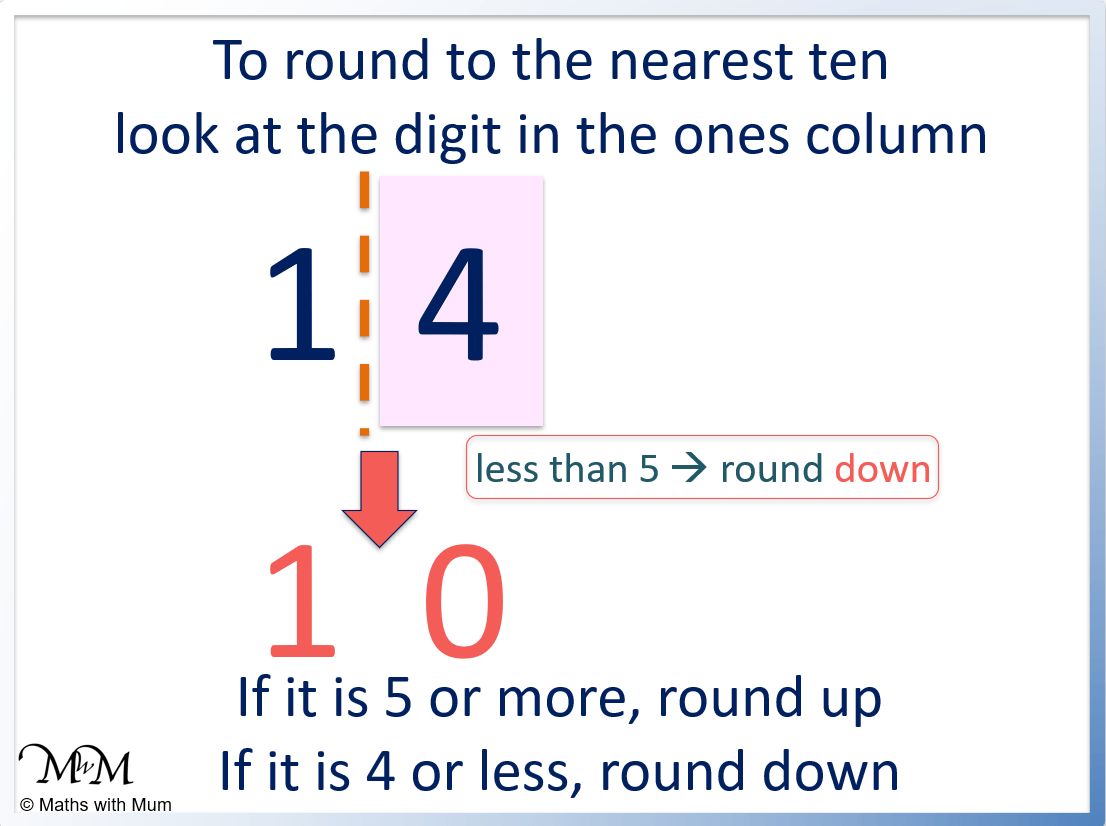For example, estimate 55 to the nearest ten. There is a 5 in the ones column and so, we round up.## How to Estimate to the Nearest Hundred To estimate a number to the nearest hundred, look at the digit in the tens column. If it is 5 or more, round up. If it is 4 or less, round down. For example, 247 rounds down to 200 because there is a 4 in the tens column.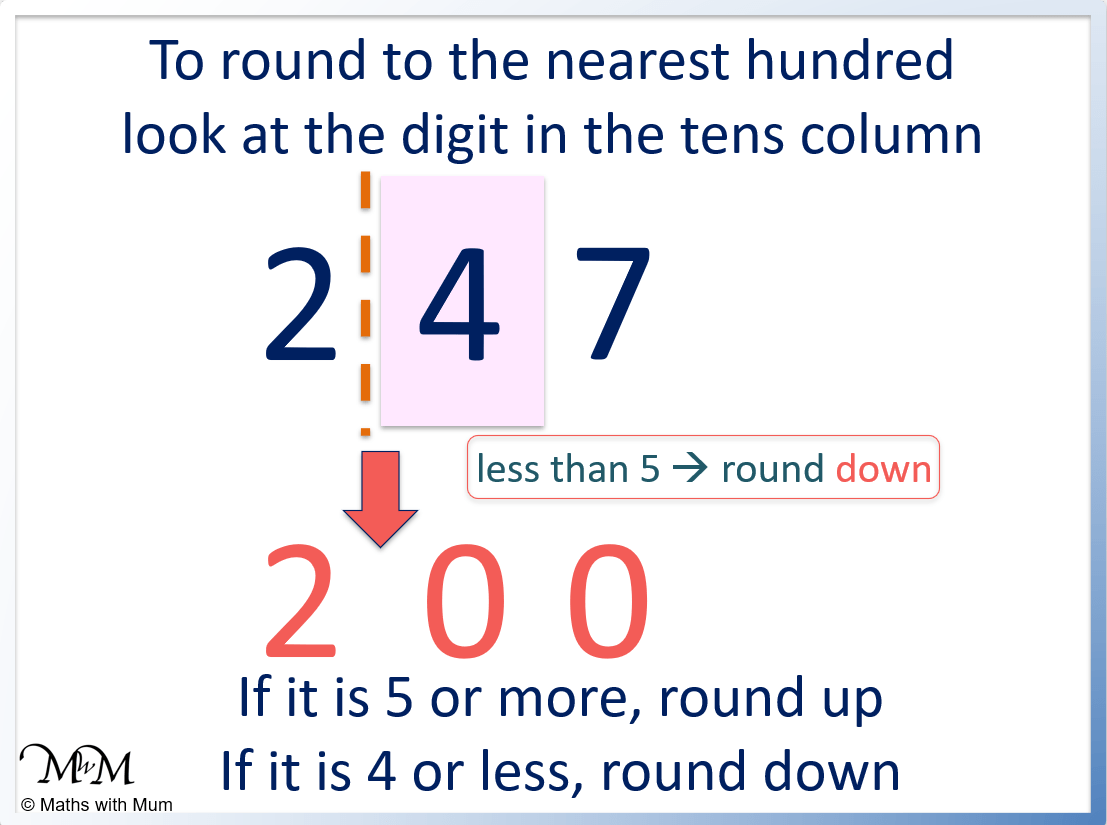For example, estimating 1363 to the nearest hundred is 1400 because 6 in the tens column is ‘5 or more’.## How to Estimate an Answer When estimating an answer use the following rules: • Focus on the digits at the start of each number as they have a greater impact on the answer. • Round each number greater than one to the nearest whole number, ten, hundred or thousand. • Round any number less than one to the nearest fractional amount. • Round all numbers before performing the calculation. For example, estimate the size of the answer to 39 × 4.85. 39 rounds up to 40. Multiples of 10 are generally easier to multiply. 4.85 rounds up to 5. Five is chosen as it is also an easier number to multiply.Since 4 × 5 = 20, 40 × 5 = 200. We need to add another zero. Here is an example of estimating the total cost of a shopping list. When estimating money, round each amount to the nearest whole number.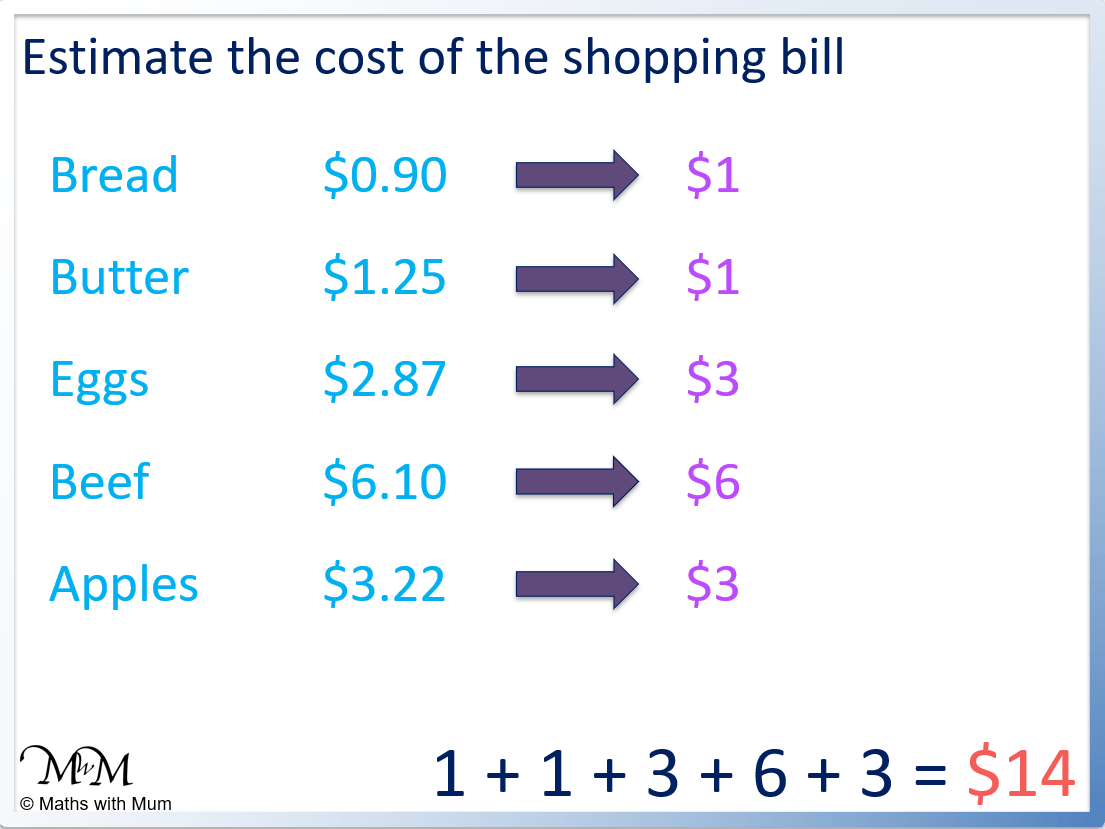•$0.90 rounds up to $1. •$1.25 rounds down to $1. •$2.87 rounds up to $3. •$6.10 rounds down to $6. •$3.22 rounds down to $3. Adding up the total we have$1 + $1 +$3 + $6 +$3 = $14. #### How to Estimate an Addition To estimate an addition, round all numbers to their first digit before adding them. To do this, look at the second digit of each number. If this digit is 5 or more, round up. If it is 4 or less, round down. For example, estimate the addition of 48 + 51. The second digit of 48 is 8, therefore we round it up to 50. The second digit of 51 is 1, therefore we round it down to 50. Perform the addition after the rounding. 50 + 50 = 100, therefore an estimate to 48 + 51 is 100.The correct answer is 99, which is only 1 off 100. Here is another example of estimating an addition. Estimate 384 + 209. The second digit of 384 is 8, therefore it rounds up to 400. The second digit of 209 is 0, therefore it rounds down to 200. 400 + 200 = 600, therefore the estimate to the addition of 384 + 209 = 600.The correct answer to 384 + 209 is 593, which is only 7 away from the estimate of 600. #### How to Estimate a Subtraction To estimate a subtraction, round each number to its first digit and then subtract. To do this, look at the second digit of each number. If it is 5 or more, round up. If it is 4 or less, round down. For example, estimate the subtraction of 73 – 29. The second digit of 73 is a 3, therefore 73 rounds down to 70. The second digit of 29 is 9, therefore 29 rounds up to 30. The estimate of 73 – 29 is 70 – 30, which equals 40.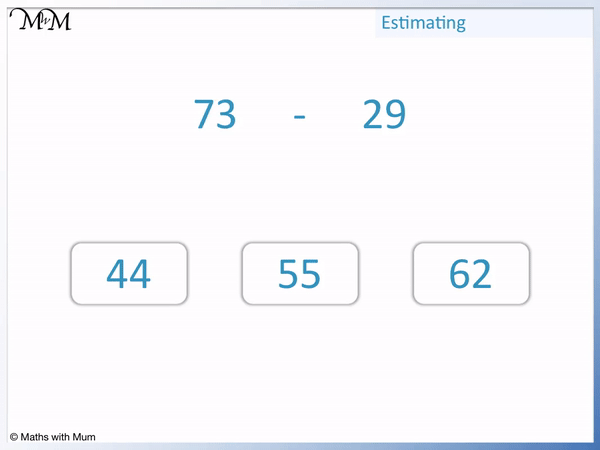The correct answer to 73 – 29 is 44, which is 4 away from the estimated answer of 40. Subtraction is used to find a difference. Find the estimated difference between 988 and 674. The second digit of 988 is 8, therefore 988 rounds up to 1000. The second digit of 674 is 7, therefore 674 rounds up to 700. 1000 – 700 = 300, therefore the estimated difference between 988 and 674 is 300.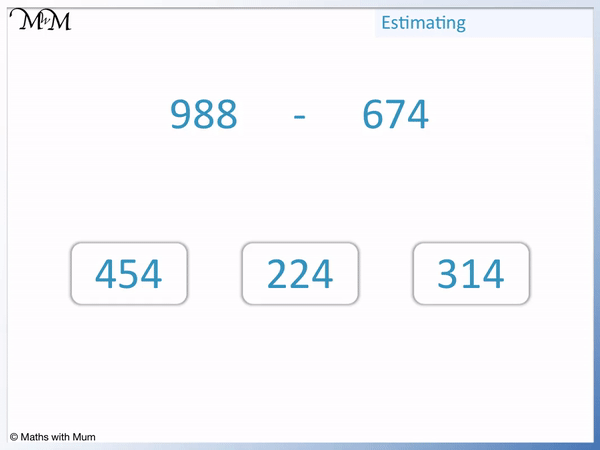The difference between 988 and 674 is 314. This is 14 away from the estimated difference of 300. #### How to Estimate Multiplication To estimate the answer to a multiplication, round the numbers to their first digit or to easy to multiply digits before multiplying them. For example, 39 × 4.85 can be estimated as 40 × 5 which equals 200. The correct answer is 189.15.Here is another example of estimating multiplication. Estimate 482 × 734. 482 can be estimated as 500. 734 can be estimated as 700.To calculate 500 × 700, multiply 5 × 7 to get 35 and add on the four zeros found in 500 and 700. 500 × 700 = 350000 and so, the estimate to 482 × 734 is 350000. The correct answer is 353788. #### How to Estimate Division To estimate division, find similar numbers that can be divided exactly. For example 15 ÷ 4 can be estimated as 16 ÷ 4 = 4. 15 is close to 16 and 16 is chosen because it can be divided exactly by 4.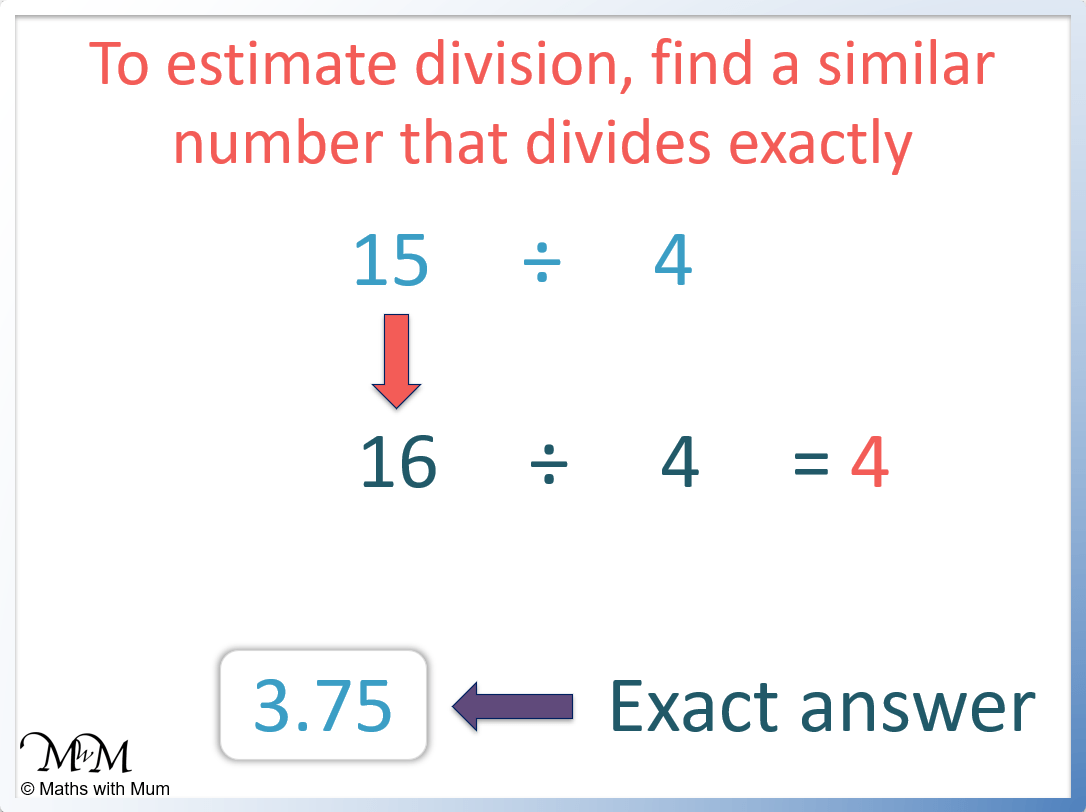For example, estimate the division 194592 ÷ 4126.In this example, 194592 rounds to 200000 and 4126 can be rounded to 4000. We can cancel the three zeros in 4000 with the three zeros in 200000 to leave 200 ÷ 4. 20 ÷ 4 = 5 and so 200 ÷ 4 = 50. Here is another example of estimating division. Estimate 19 ÷ 3. Instead of rounding 19 up to 20, it is best to round it down to 18. We choose the nearest number that can be divided exactly by 3. 18 ÷ 3 = 6 and so, 19 ÷ 3 is just a little larger than 6.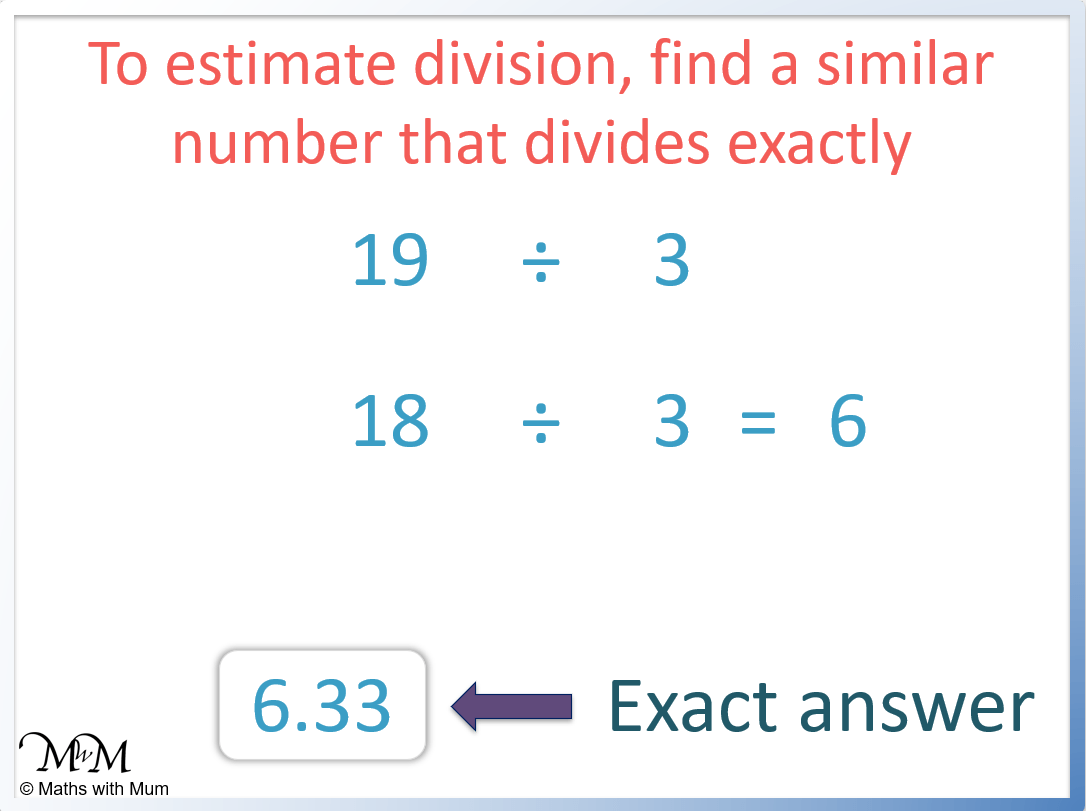19 ÷ 3 = 6.33. ## How to Estimate Decimals When calculating with decimals, try to round the decimal number to the nearest whole number. For decimals less than one whole, round the decimal to a number that is equivalent to a simple fraction. Here are some useful decimals and their fraction equivalents. Decimal Equivalent Fraction 0.1 1/10 0.2 1/5 0.25 1/4 0.33 1/3 0.4 2/5 0.5 1/2 0.6 3/5 0.66 2/3 0.75 3/4 0.8 4/5 For example, estimate 0.26 × 23.87. 0.26 can be estimated as 0.25, which is the same as 1/4. 28.87 can be estimated as 24. 0.26 × 23.87 can be estimated as 1/4 of 24 which equals 6.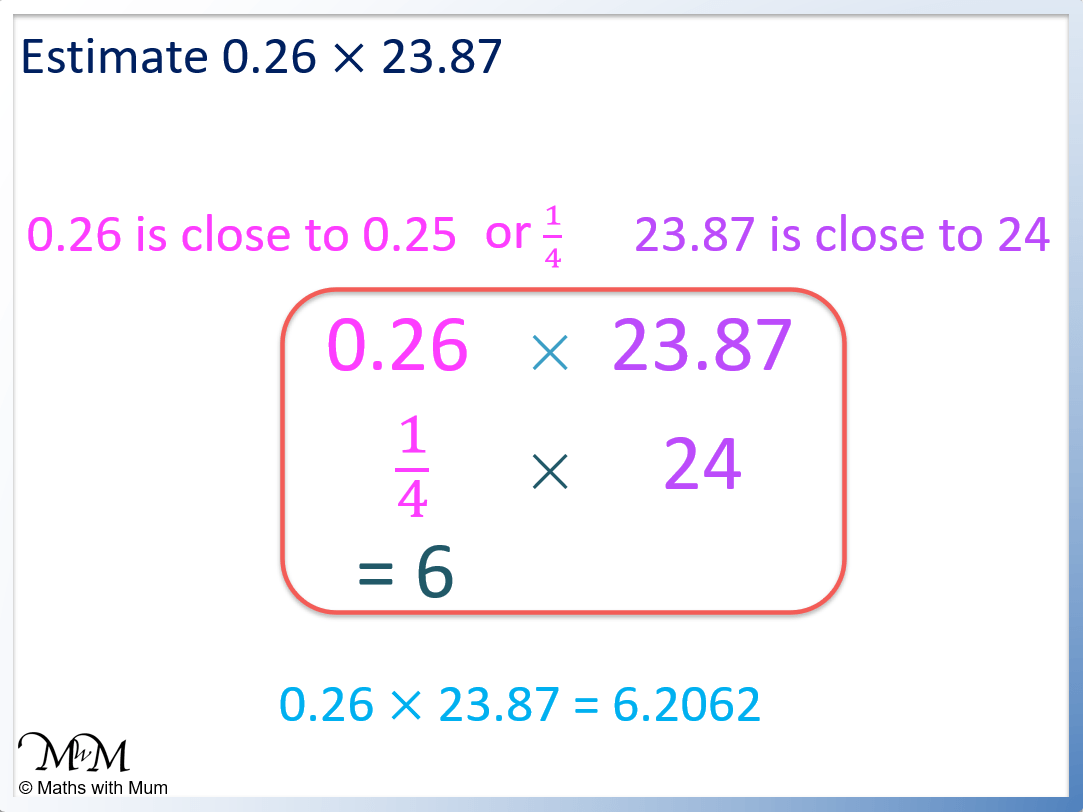#### How to Estimate Decimals When Adding To estimate addition involving decimals, round each decimal to the nearest whole number and then add them. For example, estimate 1.85 + 14.03 + 3.92.2 + 14 + 4 = 20. #### How to Estimate Decimal Subtraction To estimate subtraction involving decimals, round each decimal to the nearest whole number and then subtract them. For example, estimate 14.99 – 2.85. Rounding to the nearest whole number this can be estimated as 15 – 3 = 12.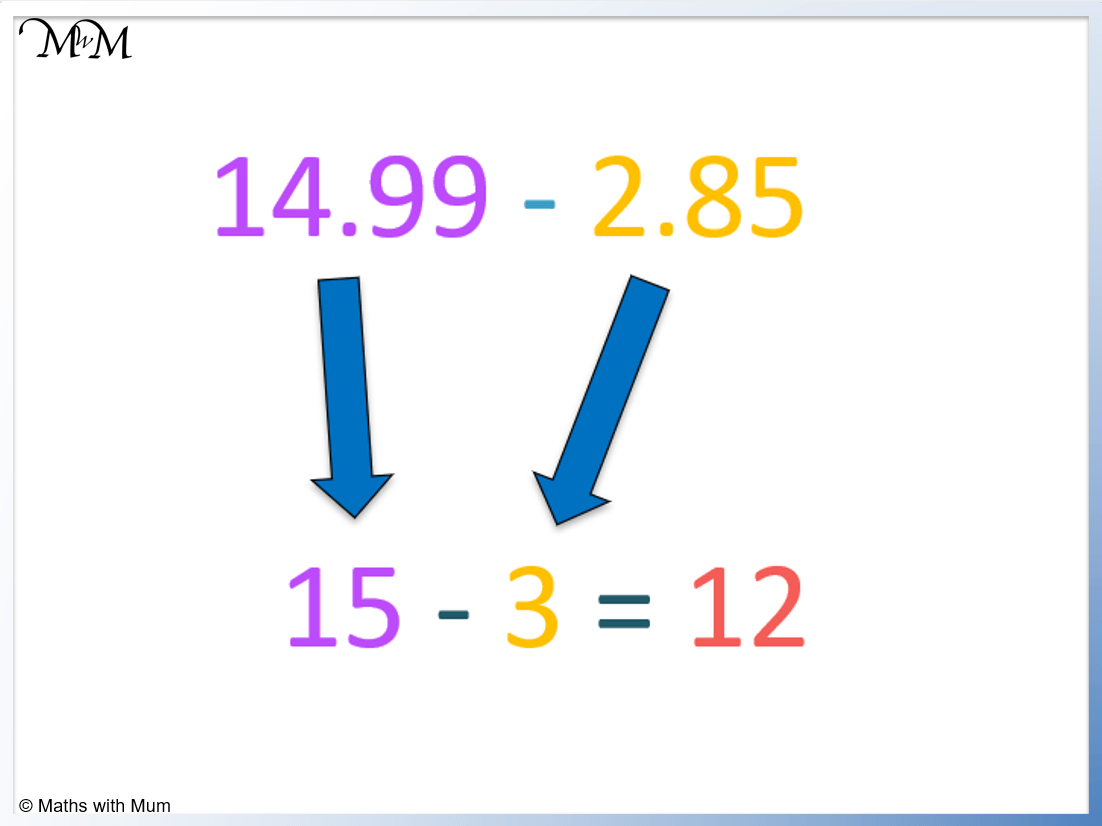#### How to Estimate Decimal Multiplication To estimate a decimal multiplication: • Round any number greater than one to the nearest whole number. • Round any decimal less than one to a decimal that has a fraction equivalent. For example estimate 0.32 × 59.3. 0.32 can be estimated as 0.33, which is equivalent to 1/3. 59.3 can be estimated as 60. 0.32 × 59.3 can be estimated to be 1/3 of 60, which equals 20.The exact answer is 18.976. #### How to Estimate Decimal Division To estimate a decimal division, round the numbers so that the division can be done exactly. For example, estimate 7.9 ÷ 2.03. The numbers can be estimated as 8 ÷ 2 and so the division can be estimated to be equal to 4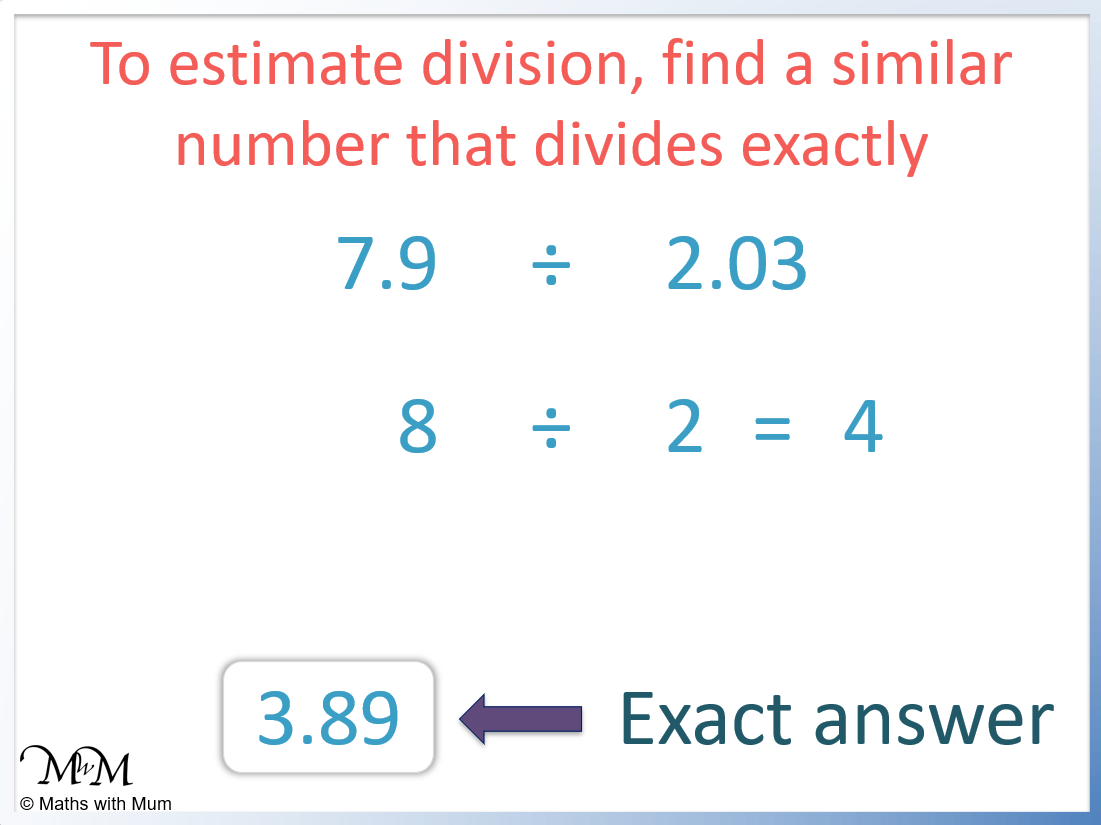The exact answer is 3.89. #### How to Estimate to the Nearest Tenth To estimate a number to the nearest tenth, look at the digit in the hundredths column. If it is 5 or more, add 1 to the digit in the tenths column. If it is 4 or less, keep the tenths digit the same. Remove all digits that follow. For example estimate 0.5814 + 2.632. There is an 8 in the hundredths column of 0.5814 and so, 0.5814 rounds up to 0.6. There is a 3 in the hundredths column of 2.632 and so, 0.2632 rounds down to 2.6. 0.6 + 2.6 = 3.2 and so the estimate for this calculation is 3.2.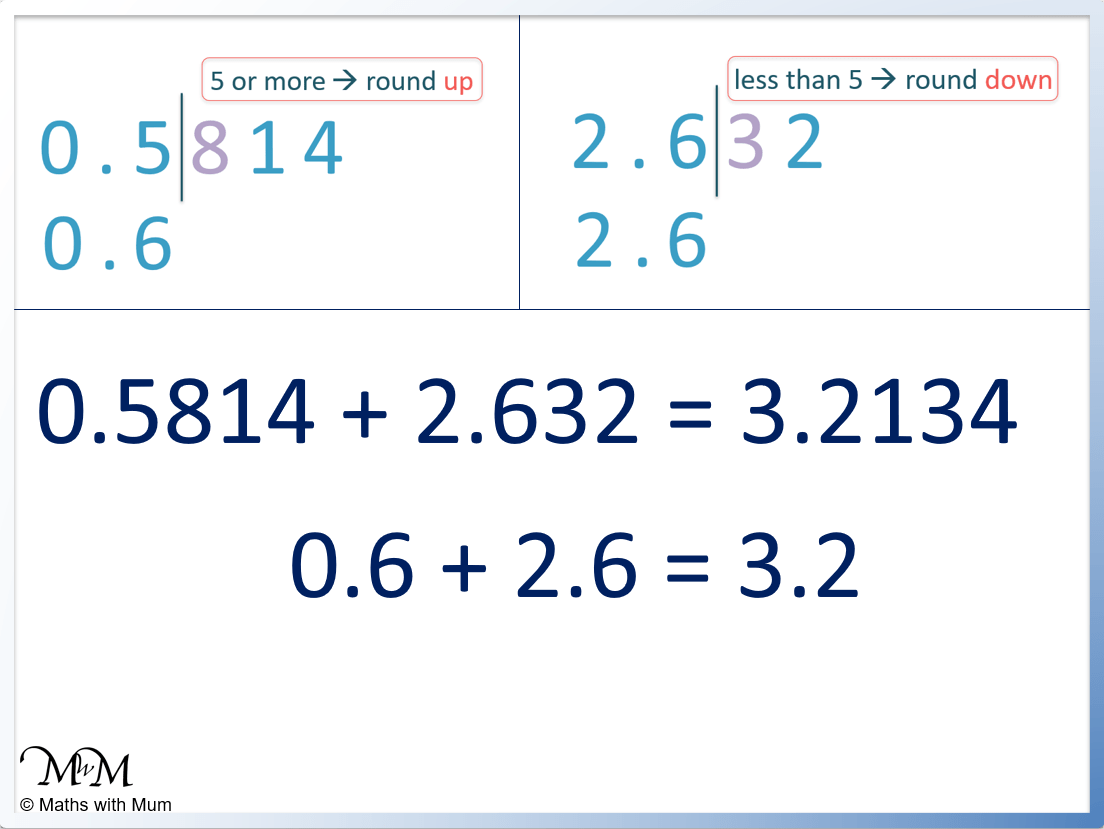The correct answer is 3.2134. ## How to Estimate Percentages To estimate a percentage: • Estimate what 50% is by dividing the total by 2. • Estimate what 10% is by dividing the total by 10. • Estimate what 5% is by finding half of 10%. • Estimate what 1% is by dividing the total by 100. • Use combinations of these percentages to find a similar percentage to that required. For example, estimate 52% of 40. 52% is very similar to 50%. Therefore an estimate of 52% of 40 will be approximately half of 40, which is 20.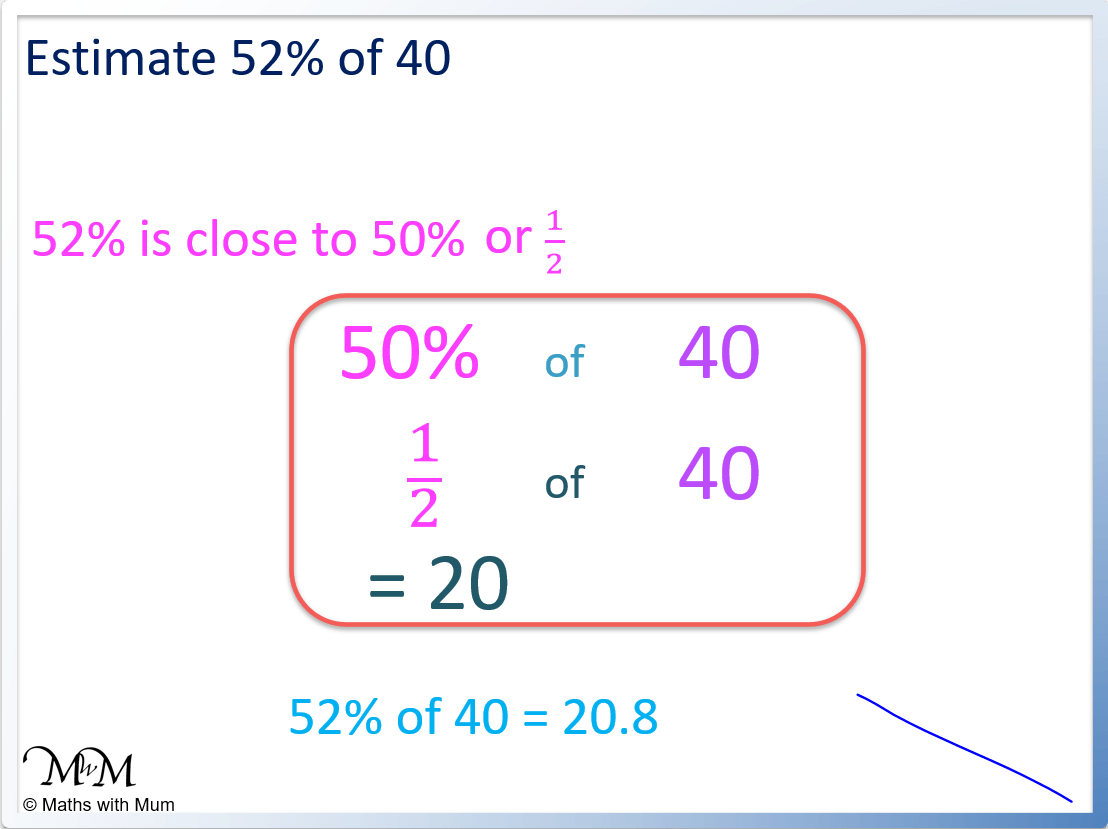We know that 52% will be a little larger than 50% and so, we can estimate it as larger than 20. 1% is found by dividing 40 by 100 to get 0.4 and so, doubling this 2% is 0.8. Therefore 52% is 20.8. For example, estimate 16% of$20.

16% is similar to 15% and so, can be estimated by adding 10% and 5%.

10% is found by dividing $20 by 10 to get$2 and then 5% is half of 10%, which is $1. 15% is therefore$2 + $1 =$3. Therefore 16% is a little larger than $3.To find 16%, add 1% to the 15% found previously. 1% is found by dividing$20 by 100 to get $0.20. Therefore 16% is$3.20.

## How to Estimate with Fractions

To estimate with fractions, round each fraction to the nearest whole number. If the fraction is less than one whole, compare the numerator to the denominator so that the fraction can be compared to a fraction that is easier to work with.

For example, estimate 14/29 of 14.

14 is exactly half of 28 and so, 14/29 can be estimated as 1/2.

1/2 of 14 is 7.#### How to Estimate Fractions When Adding

When estimating adding fractions, compare the numerator to the denominator. If the numerator is close to the denominator, estimate the fraction as one whole. If the numerator is much less than the denominator, estimate the fraction as zero. If the numerator is similar to half of the denominator, estimate the fraction as one half.

For example, estimate 5/6 + 17/20 + 5/9 + 1/11.

5/6 can be estimated as 1 whole because 5 is close to 6.

17/20 can also be estimated as 1 whole because 17 is close to 20.

5/9 can be estimated as one half because 5 is half of 10, which is close to half of 9.

1/11 can be estimated as zero since 1 is much less than 11.

Therefore 5/6 + 17/20 + 5/9 + 1/11 can be estimated as 1 + 1 + 0.5 + 0, which equals 2.5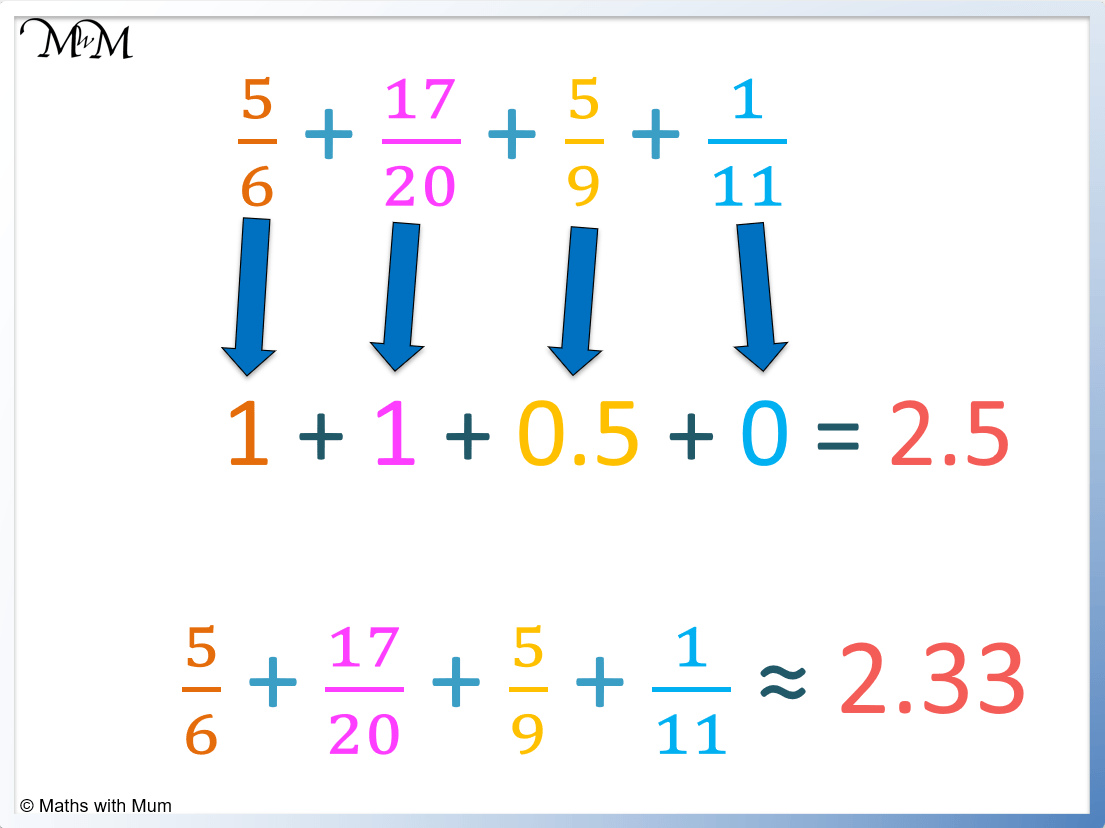The correct answer is 2.33, which is close to the estimate of 2.5.

#### How to Estimate Fractions When Subtracting

To estimate fractions when subtracting, compare the numerator to the denominator. If the numerator is close to the denominator, estimate the fraction as one whole. If the numerator is much less than the denominator, estimate the fraction as zero. If the numerator is similar to half of the denominator, estimate the fraction as one half.

For example, estimate 37/86/11.

7/8 can be estimated as one whole since 7 is close to 8. Therefore 37/8 can be estimated as 4.

6/11 can be estimated as 1/2 since 6 is close to half of 11.

Therefore 37/86/11 can be estimated as 4 – 0.5 which equals 3.5.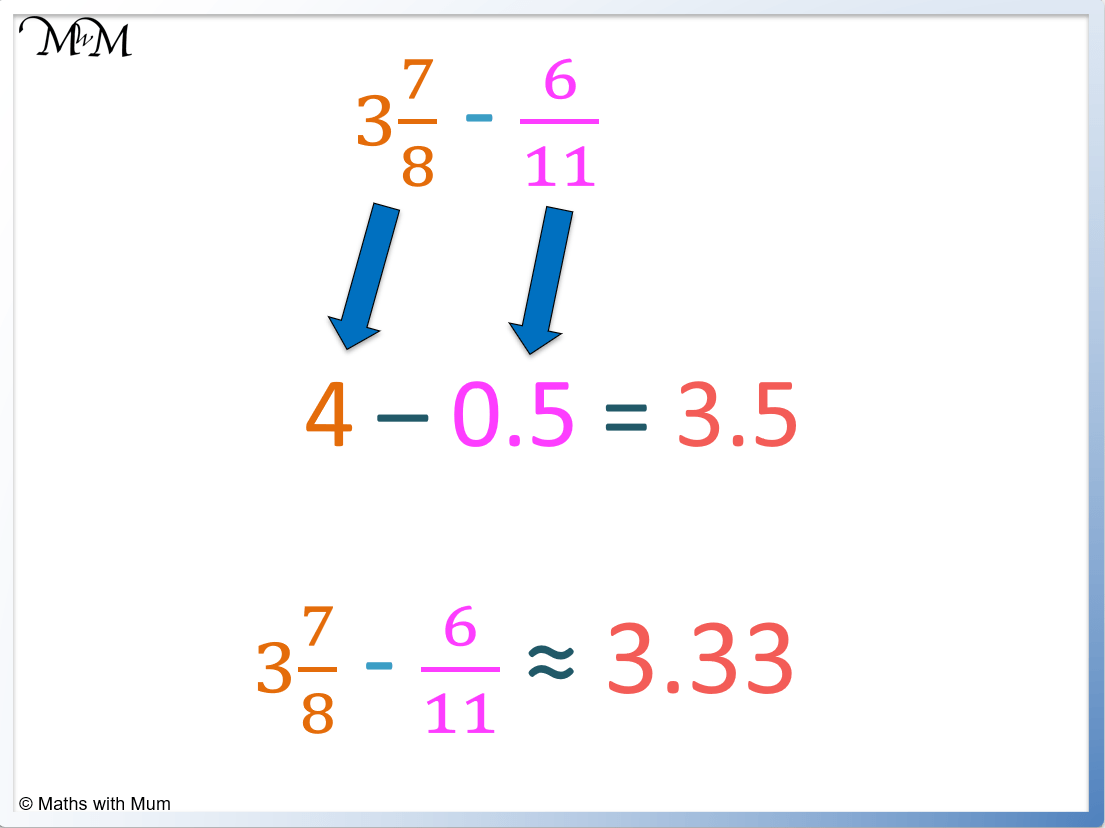The correct answer is approximately 3.33 which is close to the estimate of 3.5.

#### How to Estimate Fractions When Multiplying

To estimate multiplication with fractions, compare each fraction to one half. If the fraction is greater than or equal to one half, round it up to the next whole number. If the fraction is less than one half, round it down to the previous whole number. Then multiply the whole numbers together.

For example, estimate 44/5 × 21/4.

44/5 can be estimated as 5 since 4/5 is larger than 1/2.

21/4 can be estimated as 2 since 1/4 is less than 1/2.

44/5 × 21/4 can be estimated as 5 × 2 = 10.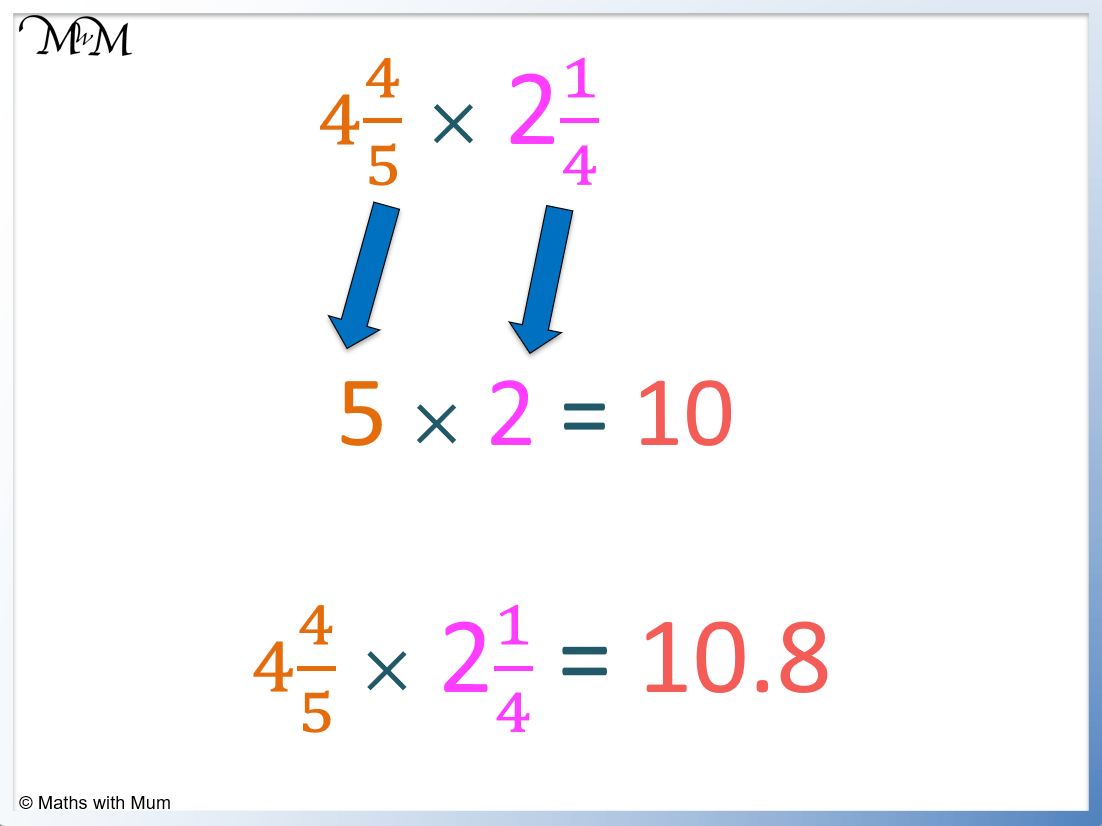The exact answer is 10.8 which is close to the estimate of 10.

#### How to Estimate Fractions When Dividing

To estimate division with fractions, compare each fraction to one half. If the fraction is greater than or equal to one half, round it up to the next whole number. If the fraction is less than one half, round it down to the previous whole number. Then divide the whole numbers.

For example, estimate 33/4 ÷ 21/5.

33/4 can be estimated as 4 since 3/4 is larger than one half.

21/5 can be estimated as 2 since 1/5 is less than one half.

33/4 ÷ 21/5 can be estimated as 4 ÷ 2 which equals 2.The correct answer is approximately 1.70 which is close to the estimate of 2.Now try our lesson on Halving Odd Numbers where we learn how to halve an odd number.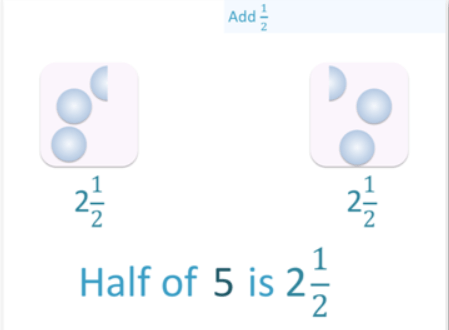error: Content is protected !!